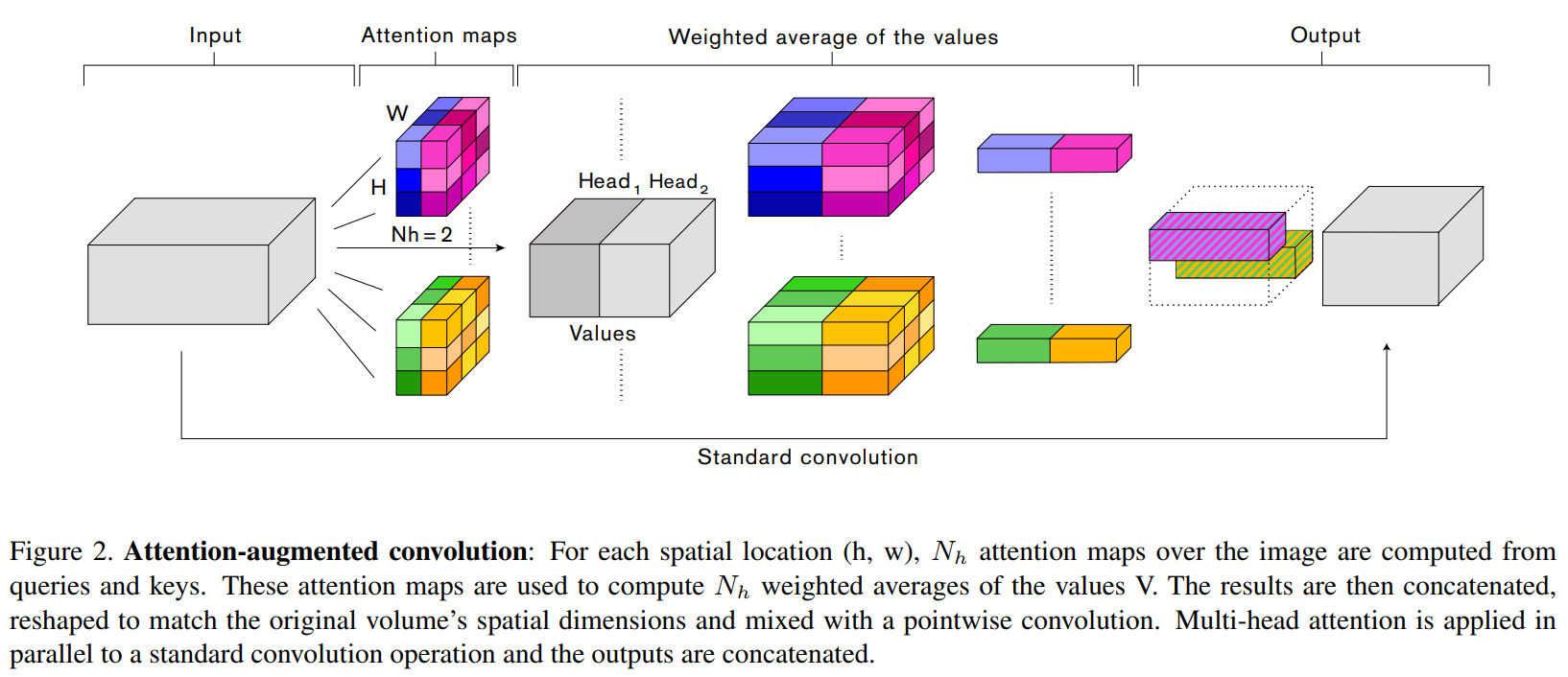Last Commit
May. 23, 2019
Created
Apr. 23, 2019

# Implementing Attention Augmented Convolutional Networks using Pytorch

• In the paper, it is implemented as Tensorflow. So I implemented it with Pytorch.

## Update (2019.05.11)

• Fixed an issue where key_rel_w and key_rel_h were not found as learning parameters when using relative=True mode.

• In "relative = True" mode, you can see that "key_rel_w" and "key_rel_h" are learning parameters. In "relative = False" mode, you do not have to worry about the "shape" parameter.

• Example, relative=True, stride=1, shape=32

```import torch

from attention_augmented_conv import AugmentedConv

use_cuda = torch.cuda.is_available()
device = torch.deivce('cuda' if use_cuda else 'cpu')

tmp = torch.randn((16, 3, 32, 32)).to(device)
augmented_conv1 = AugmentedConv(in_channels=3, out_channels=20, kernel_size=3, dk=40, dv=4, Nh=4, relative=True, stride=1, shape=32).to(device)
conv_out1 = augmented_conv1(tmp)
print(conv_out1.shape) # (16, 20, 32, 32)

for name, param in augmented_conv1.named_parameters():
print('parameter name: ', name)```
• As a result of parameter name, we can see "key_rel_w" and "key_rel_h".

• Example, relative=True, stride=2, shape=16

```import torch

from attention_augmented_conv import AugmentedConv

use_cuda = torch.cuda.is_available()
device = torch.deivce('cuda' if use_cuda else 'cpu')

tmp = torch.randn((16, 3, 32, 32)).to(device)
augmented_conv1 = AugmentedConv(in_channels=3, out_channels=20, kernel_size=3, dk=40, dv=4, Nh=4, relative=True, stride=2, shape=16).to(device)
conv_out1 = augmented_conv1(tmp)
print(conv_out1.shape) # (16, 20, 16, 16)```
• This is important, when using the "relative = True" mode, the stride * shape should be the same as the input shape. For example, if input is (16, 3, 32, 32) and stride = 2, the shape should be 16.

## Update (2019.05.02)

• I have added padding to the "AugmentedConv" part.

• You can use it as you would with nn.conv2d.

• I will attach the example below as well.

• Example, relative=False, stride=1

```import torch

from attention_augmented_conv import AugmentedConv

use_cuda = torch.cuda.is_available()
device = torch.deivce('cuda' if use_cuda else 'cpu')

temp_input = torch.randn((16, 3, 32, 32)).to(device)
augmented_conv = AugmentedConv(in_channels=3, out_channels=20, kernel_size=3, dk=40, dv=4, Nh=1, relative=False, stride=1).to(device)
conv_out = augmented_conv(tmp)
print(conv_out.shape) # (16, 20, 32, 32), (batch_size, out_channels, height, width)```
• Example, relative=False, stride=2
```import torch

from attention_augmented_conv import AugmentedConv

use_cuda = torch.cuda.is_available()
device = torch.deivce('cuda' if use_cuda else 'cpu')

temp_input = torch.randn((16, 3, 32, 32)).to(device)
augmented_conv = AugmentedConv(in_channels=3, out_channels=20, kernel_size=3, dk=40, dv=4, Nh=1, relative=False, stride=2).to(device)
conv_out = augmented_conv(tmp)
print(conv_out.shape) # (16, 20, 16, 16), (batch_size, out_channels, height, width)```
• I added an assert for parameters (dk, dv, Nh).
```assert self.Nh != 0, "integer division or modulo by zero, Nh >= 1"
assert self.dk % self.Nh == 0, "dk should be divided by Nh. (example: out_channels: 20, dk: 40, Nh: 4)"
assert self.dv % self.Nh == 0, "dv should be divided by Nh. (example: out_channels: 20, dv: 4, Nh: 4)"
assert stride in [1, 2], str(stride) + " Up to 2 strides are allowed."```

## I posted two versions of the "Attention-Augmented Conv"

• Paper version is here
• AA-Wide-ResNet version is here

# Reference

## Method## Experiments

• In the paper, they said that We augment the Wide-ResNet-28-10 by augmenting the first convolution of all residual blocks with relative attention using Nh=8 heads and κ=2, υ=0.2 and a minimum of 20 dimensions per head for the keys.
Datasets Model Accuracy Epoch Training Time
CIFAR-10 Wide-ResNet 28x10(WORK IN PROCESS)
CIFAR-100 Wide-ResNet 28x10(WORK IN PROCESS)
CIFAR-100 Just 3-Conv layers(channels: 64, 128, 192) 61.6% 100 22m
CIFAR-100 Just 3-Attention-Augmented Conv layers(channels: 64, 128, 192) 59.82% 35 2h 23m

## Requirements

• tqdm==4.31.1
• torch==1.0.1
• torchvision==0.2.2